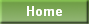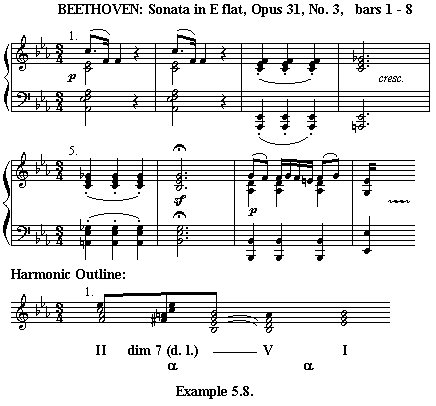CHAPTER 5 (PART 4) EXTENSIONS WITHIN THE BASIC STRUCTURE The Dynamic Introduction Sometimes the opening section of the musical phrase is subdivided into two syntactic elements. The initial static harmony is then preceded by a dynamic chord progression that I will refer to as a dynamic introduction. This is a kind of extended upbeat or lead-in to the static harmony. It can be present in the first phrase of a piece of music or any subsequent phrase. This type of phrase extension is less common than the previous two types explained above. All the techniques explained in chapter 2 for creating dynamic harmony can be applied. These are: The use of strong α, β, and γ chord progressions, The addition of essential sevenths and sharpening of minor 3rds to strengthen the harmonic movement. The use of passing notes and passing chords filling in between functional chords. The use of linear progressions filling in between functional chords. Dynamic introductions end with a dominant chord that moves to the initial tonic chord of the static harmony. The final dominant chord can be extended into a dominant prolongation. Thus the form of dynamic introduction is similar to a structure of a complete closing section but with the final tonic chord overlapping with the first tonic chord of the static harmony. For dynamic harmony at the start of a phrase to be a Dynamic Introduction it must be fully integrated or internal to the phrase. Dynamic harmony may also exist as independent lead-ins to phrases either as introductions or as linking passages. In the latter case the dynamic introduction is an incomplete phrase and is external to the complete phrase. (See Chapter 6.) The following figure shows the form of a complete phrase extended by a dynamic introduction:The first subject of the Beethoven Piano Sonata, Opus 31 No. 3. starts with a dynamic introduction:This dynamic introduction is based on a simple II - V - I progression. This is elaborated by a cadential 6 4 appoggiatura chord and a passing dominant leading diminished seventh chord. After an ascending scale passage, the dynamic introduction repeats and the static harmony starts at bar 17 and continues to bar 27 where the dynamic harmony of the closing section starts. The dynamic introduction belongs to the same phrase as the static harmony in bars 17 to 27 as both syntactic elements contain the similar motivic material (the descending 5th dotted figure) and are both part of the first subject. In this example, the dynamic introduction is an integral part of the syntactic phrase. To avoid any confusion it should be made clear that there is no relationship between a dynamic introduction and a formal introduction. A dynamic introduction is a syntactic element and performs a syntactic function in a musical phrase. The only purpose of the expression dynamic introduction is to explain how the harmony functions in relation to the musical phrase. It can exist at the start of any phrase in a piece of music not necessarily the first phrase. A formal introduction may contain whole syntactic phrases. Sometimes a dynamic introduction may precede a phrase without being integrated within the phrase. This type of external phrase extension will be dealt with in Chapter 6. Demo 3 is an example of a full phrase containing a dynamic introduction although the dynamic introduction here consists of only a single dominant chord extended over two bars. The full book will contain further examples of the dynamic introduction. Next Chapter